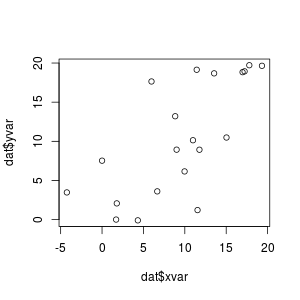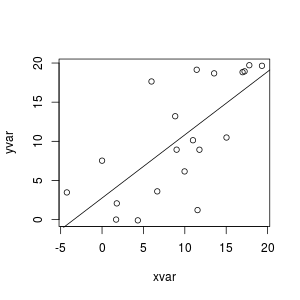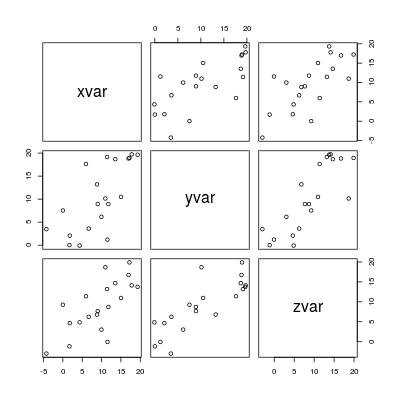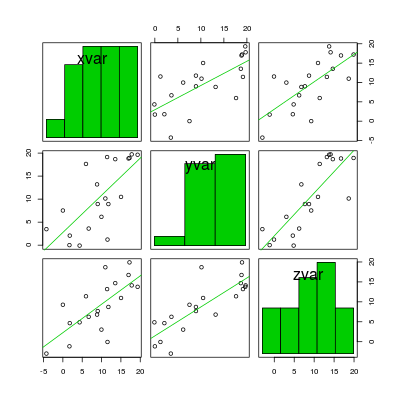## Problem

You want to make a scatterplot.

## Solution

``````set.seed(955)
# Make some noisily increasing data
dat <- data.frame(xvar = 1:20 + rnorm(20,sd=3),
yvar = 1:20 + rnorm(20,sd=3),
zvar = 1:20 + rnorm(20,sd=3))

#>        xvar         yvar        zvar
#> 1 -4.252354  3.473157275 -2.97806724
#> 2  1.702318  0.005939612 -1.16183118
#> 3  4.323054 -0.094252427  4.85516658
#> 4  1.780628  2.072808278  4.65078709
#> 5 11.537348  1.215440358 -0.06613962
#> 6  6.672130  3.608111411  6.24349897
``````

### Basic scatterplots

``````# Plot the points using the vectors xvar and yvar
plot(dat\$xvar, dat\$yvar)

# Same as previous, but with formula interface
plot(yvar ~ xvar, dat)

fitline <- lm(dat\$yvar ~ dat\$xvar)
abline(fitline)
``````### Scatterplot matrices

It is also possible to make a matrix of scatterplots if you would like to compare several variables.

See this for a way to make a scatterplot matrix with r values.

``````# A scatterplot matrix
plot(dat[,1:3])

# Another way of making a scatterplot matrix, with regression lines
# and histogram/boxplot/density/qqplot/none along the diagonal
library(car)
scatterplotMatrix(dat[,1:3],
diagonal="histogram",
smooth=FALSE)
``````To calculate the corresponding correlation matrix, see ../../Statistical analysis/Regression and correlation.

To visualize the correlation matrix, see ../Correlation matrix.